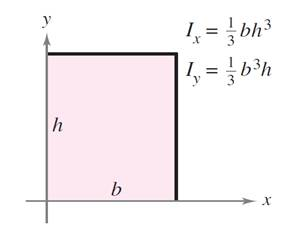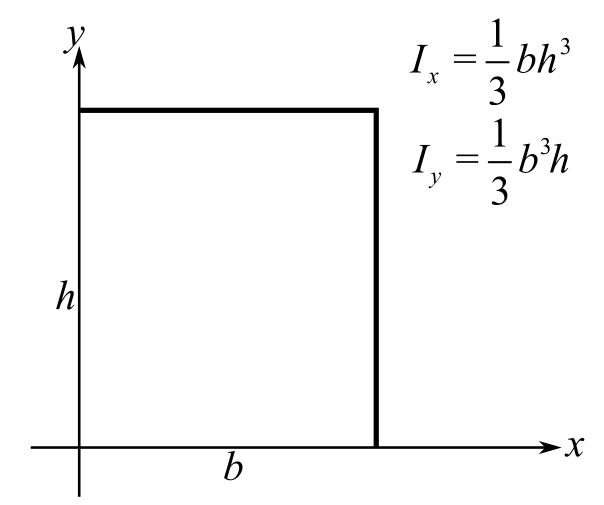Chapter 14.4, Problem 29E

Chapter
Section
Textbook Problem

Finding the Radius of Gyration About Each Axis in Exercises 29-34, verify the given moment(s) of inertia and find x ¯ ¯ and y ¯ ¯ . Assume that each lamina has a density of ρ = 1 gramper square centimeter. (These regions are common shapes use din engineering.)RectangleTo determine

To calculate: The values of x¯¯ and y¯¯ and also, verify the moment of inertia of the provided lamina.Explanation

Given:

The given lamina is shown in the figure below:

Assume the density of lamina as ρ=1.

Formula Used:

The moment of inertia about x – axis is determined by the formula, Ix=0b0hy2dydx.

The moment of inertia about y – axis is determined by the formula, Iy=0b0hx2dydx.

Calculation:

First, calculate the mass of the provided lamina as follows,

m=0b0hdydx=0b[y]0hdx=0bhdx=[hx]0b

This gives,

m=bh

The moment of inertia about x-axis is calculated as shown below:

Ix=0b0hy2dydx=0b[y33]0hdx=0bh33dx=h33[x]0b

Then,

Ix=bh33

This shows moment of inertia about x-axis is equal to the provided value moment of inertia

Still sussing out bartleby?

Check out a sample textbook solution.

See a sample solution

The Solution to Your Study Problems

Bartleby provides explanations to thousands of textbook problems written by our experts, many with advanced degrees!

Get Started

23-30 Find the limit. limxex2

Calculus (MindTap Course List)

Explain why 2004 nickels are worth more than 100.

Mathematical Excursions (MindTap Course List)

Solve each equation and check: 8y=3

Elementary Technical Mathematics

In Exercises 1-8, determine which of the matrices are regular. [10.800.2]

Finite Mathematics for the Managerial, Life, and Social Sciences